# Check if Cell Contains Any Number – Excel & Google Sheets

This tutorial demonstrates how to check if a cell contains any number in Excel and Google Sheets.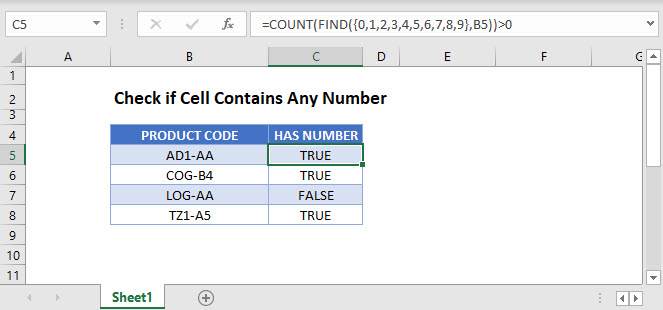## Cell Contains Any Number

In Excel, if a cell contains numbers and letters, the cell is considered a text cell. You can check if a text cell contains any number by using the COUNT and FIND Functions.

``=COUNT(FIND({0,1,2,3,4,5,6,7,8,9},B3))>0``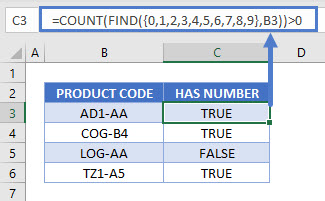The formula above checks for the digits 0–9 in a cell and counts the number of discrete digits the cell contains. Then it returns TRUE if the count is positive or FALSE if it is zero.

Let’s step through each function below to understand this example.

### FIND a Number in a Cell

First, we use the FIND Function. The FIND Function finds the position of a character within a text string.

``=FIND({0,1,2,3,4,5,6,7,8,9},B3)``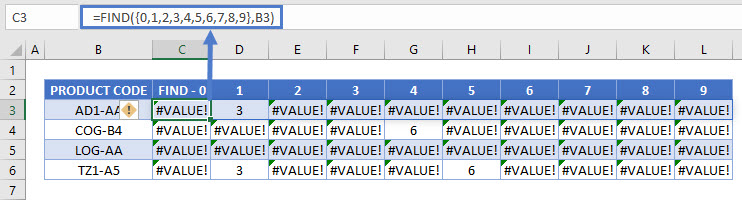In this example, we use an array of all numerical characters (digits 0–9) and find each one in the cell. Since our input is an array – in curly brackets {} – our output is also an array. The example above shows how the FIND Function is performed ten times on each cell (once for each numerical digit).

If the number is found, it’s position is output. Above you can see the number “1” is found in the 3rd position in the first row and “4” is found in the 6th position in the 2nd row.

Note: The FIND and SEARCH Functions return the same result when used to search for numbers. Either function can be used.

### COUNT the Number of Digits

Next, we count the non-error outputs from the last step. The COUNT Function counts the number of numeric values found in the array, ignoring errors.

``=COUNT(C3:L3)``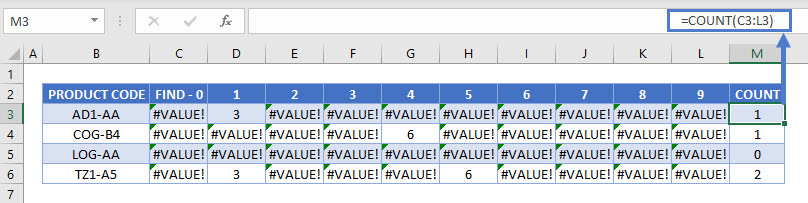### Test the Number Count

Finally, we need to test whether the result from the last step is greater than zero. The formula below returns TRUE for non-zero counts (where the target cell contains a number) and FALSE for any zero counts.

``=M3>0``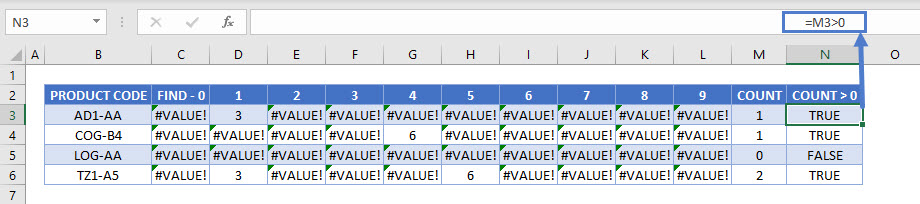Combining these steps gives us our initial formula:

``=COUNT(FIND({0,1,2,3,4,5,6,7,8,9},B3))>0``## Check if Cell Contains Specific Number

To check if a cell contains a specific number, we can use the FIND or SEARCH Function.

``=FIND(5,B3)``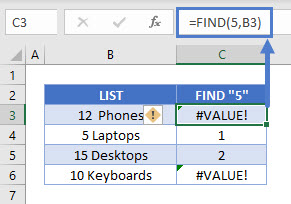In this example we use the FIND Function to check for the number 5 in column B. It returns the position of the number 5 in the cell if it is found and a VALUE error if “5” is not found.

## Check if Cell Contains Any Number – Google Sheets

These formulas work the same in Google Sheets as in Excel. However, you need to press CTRL + SHIFT + ENTER for Google Sheets to recognize an array formula.

Alternatively, you could type “ArrayFormula” and put the formula in parentheses. Both methods produce the same result.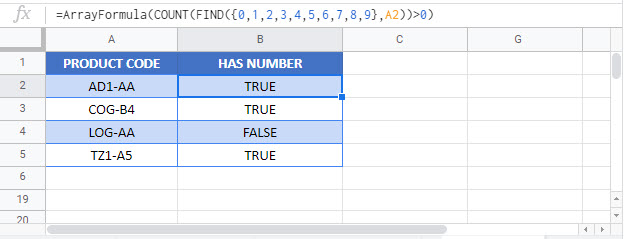### Excel Practice Worksheet

Practice Excel functions and formulas with our 100% free practice worksheets!